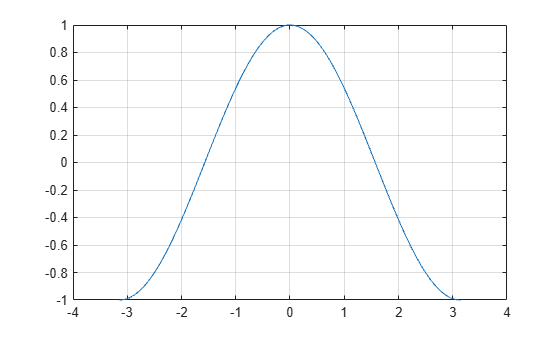# cos

## Syntax

``Y = cos(X)``

## Description

````Y = cos(X)` returns the cosine for each element of `X`. The `cos` function operates element-wise on arrays. The function accepts both real and complex inputs. For real values of `X`, `cos(X)` returns real values in the interval [-1, 1].For complex values of `X`, `cos(X)` returns complex values. ```

## Examples

collapse all

Plot the cosine function over the domain $-\pi \le x\le \pi$.

```x = -pi:0.01:pi; plot(x,cos(x)) grid on```Calculate the cosine of the complex angles in vector `x`.

```x = [-i pi+i*pi/2 -1+i*4]; y = cos(x)```
```y = 1×3 complex 1.5431 + 0.0000i -2.5092 - 0.0000i 14.7547 +22.9637i ```

## Input Arguments

collapse all

Input angle in radians, specified as a scalar, vector, matrix, or multidimensional array.

Data Types: `single` | `double`
Complex Number Support: Yes

## Output Arguments

collapse all

Cosine of input angle, returned as a real-valued or complex-valued scalar, vector, matrix or multidimensional array.

collapse all

### Cosine Function

The cosine of an angle, α, defined with reference to a right angled triangle isThe cosine of a complex argument, α, is

`$\text{cos}\left(\alpha \right)=\frac{{e}^{i\alpha }+{e}^{-i\alpha }}{2}\text{\hspace{0.17em}}.$`